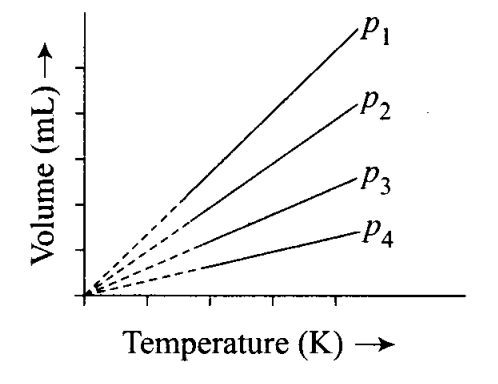# A plot of volume (V) versus temperature (T)`
Question:

A plot of volume (V) versus temperature (T) for a gas at constant pressure is a straight line passing through the origin.

The plots at different values of pressure are shown in the figure. Which , of the following order of pressure is correct for this gas?(a)P1 >P2 >P3 >P4

(b) P1 =p2 =p3 =p4

(c) P1 23

(d) px 2=p34

Solution:

(c) At a particular temperature pV= constant.

Thus,

P1V1 =P2V2=P3V3=P4V4

As V1> V2 > V3 > V 4, therefore, P1 < P< P4.